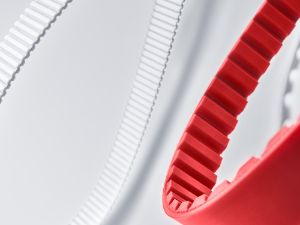# 动力传动用 APSOdrive® 皮带

## 可满足最高性能要求的橡胶带和聚氨酯同步带

••• Low maintenance applications
• Low noise applications
• High shock applications
• High vibration applications

### CONTI® SYNCHROCHAIN

CONTI® SYNCHROCHAIN 实现了高抗撕裂性和卓越拉伸强度的结合，使其成为当今世界上性能最好的同步带之一。此款含 CTD 齿型的高性能同步带让极端情境下的应用成为了可能。此款具备高减振能力的皮带设计用于具有极高加速力的传动装置，以及在低速时可靠地传输高扭矩。为应对突然加减速时产生的冲击载荷，CONTI® SYNCHROCHAIN 采用了一种高抗拉伸和耐撕裂的复合材料。这种复合材料能够可靠地吸收最大的浪涌，并保证脉冲传动装置在连续工作中免维护地运行。

### BRECOFLEXmove®

BRECOFLEXmove® 是一种专门为高性能且有着极高刚度要求的牵引传动装置设计的同步带。新开发的钢丝线芯提高了皮带的刚度和抗撕裂性，让传输更强的力成为了可能。通过有限元法 (FEM) 优化了齿面的几何形状，从而优化了接触压力分布，减少了同步带与法兰之间的摩擦，从而最大限度地减少了磨损。此外，还在齿面涂上经过摩擦力优化的层状涂布。

## 产品表

         BRECOflex® 完全闭口型同步带 皮带牙齿与轮齿啮合 聚氨酯 产品名称 产品说明 类型  牵引传动 材料 可用型号 优势 重要应用领域 订购 单面 双面 背部有加固皮带 带运输涂层 齿侧尼龙面 背侧尼龙面 提高动力传输率 精准定位 用于反向弯曲/反向张紧惰轮 抗老化和耐臭氧 耐油、耐脂 高环境温度（高于 +80°C） 低维护应用 低噪音应用 高冲击应用 高振动应用 x x x x x x x x x (x) (x) x x x BRECO-V® 焊接型同步带 皮带牙齿与轮齿啮合 聚氨酯 x x x x x x x x (x) (x) x x BRECOmove® 高性能同步带 皮带牙齿与轮齿啮合 聚氨酯 x x x x (x) (x) x x BRECOclassic® 替代标准型同步带 皮带牙齿与轮齿啮合 聚氨酯 x x x x x x (x) (x) (x) x Continental Synchroflex® 完全闭口型同步带 皮带牙齿与轮齿啮合 聚氨酯 x (x) x x (x) (x) x x Continental Synchroflex GenIII® 高性能同步带 皮带牙齿与轮齿啮合 聚氨酯 x x x x (x) (x) x x x 链 产业链 皮带牙齿与轮齿啮合 金属 x x x x x Continental SilentSync® 自导式高性能同步带 皮带牙齿与轮齿啮合 橡胶 x x x (x) x x x x x Continental Synchrobelt® 完全闭口型同步带 皮带牙齿与轮齿啮合 橡胶 x x x (x) x x Continental Synchroforce CXP®/CXA® 性能先进的完全闭口型同步带 皮带牙齿与轮齿啮合 橡胶 x x x x (x) x x x Continental Synchroforce Carbon 高性能完全闭口型同步带 皮带牙齿与轮齿啮合 橡胶 x x x x x x x x x Continental Synchrotwin® 双面同步带 皮带牙齿与轮齿啮合 橡胶 x x x (x) x x Continental Synchrochain® 高性能完全闭口型同步带 皮带牙齿与轮齿啮合 聚氨酯 x x x x x (x) x x Continental Synchrochain Carbon® 高性能完全闭口型同步带 皮带牙齿与轮齿啮合 聚氨酯 x x x x x (x) x x 包布V带 包布V带 摩擦 橡胶 x x x x (x) x x x 切割带 切割带 摩擦 橡胶 x x x (x) (x) x x x 联组V带 联组V带 摩擦 橡胶 x x x (x) x x x 多楔带 多楔带 摩擦 橡胶 x x (x) x x x

### 聚氨酯

• 钢丝绳带来的长度稳定性和低拉伸性
• 抗变形和高剪切强度
• 按客户要求自定义的皮带轮齿型公差
• 可提供自导式传动带
• 高定位精度
• 可提供自定义解决方案

• 可提供各种芯线
- 高弹性
- 高功率
- 有不锈钢芯线材质
- 有芳纶材质
• 高节距精度
• 可行的定制化二次加工
- 涂层
- 挡块（焊接或螺丝固定）
- 机械加工
• 可提供特殊的聚氨酯复合材料

• 良好的阻尼能力
• 低侧向力
• 低噪音
• 低跳齿倾向
• 可提供防静电版
• 卓越的性价比

• 高性能复合材料
- 卓越的耐油性
- 耐高温
• 聚四氟乙烯加强齿面织布
• 涂层